# Convert Time to Decimal (or Decimal to Time) – Excel & Google Sheets

This tutorial will demonstrate how to convert time values to decimal values (or vis-a-versa) in Excel and Google Sheets.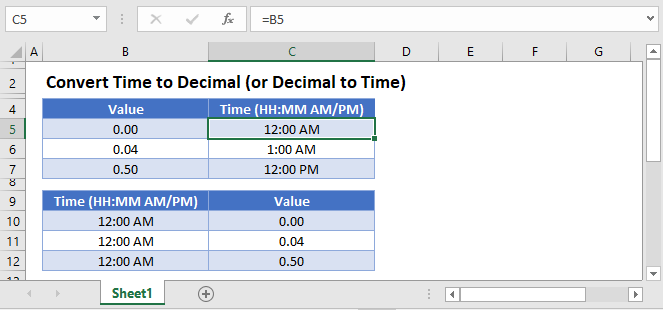## Decimal to Time

In Excel, times are stored as decimal values where each 1/24th represents one hour: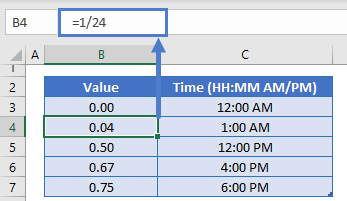To convert a decimal value to time, all you need to do is adjust the Number Formatting to Time: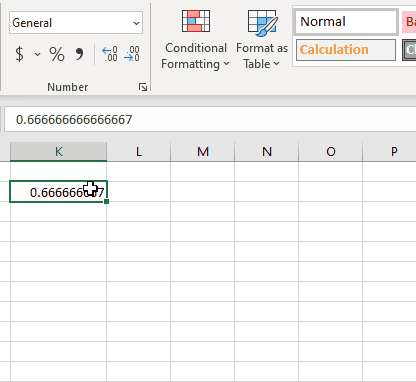## Time to Decimal

If you have a time value, all you need to do is change the Number Formatting to General to see the decimal value: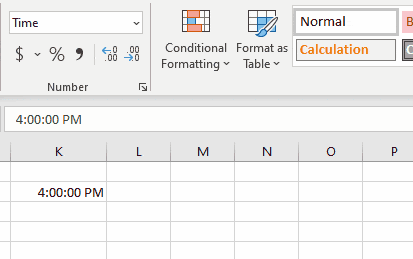If the time is stored as text, first you’ll need to use the TIMEVALUE Function before converting to decimal format:

``=TIMEVALUE(B3)``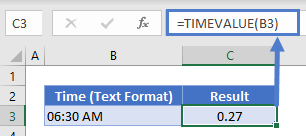## Times & Decimals in Google Sheets

All of the above examples work exactly the same in Google Sheets as in Excel.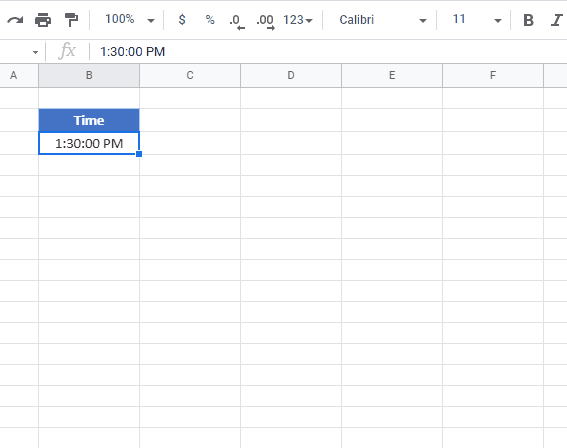### Excel Practice Worksheet

Practice Excel functions and formulas with our 100% free practice worksheets!